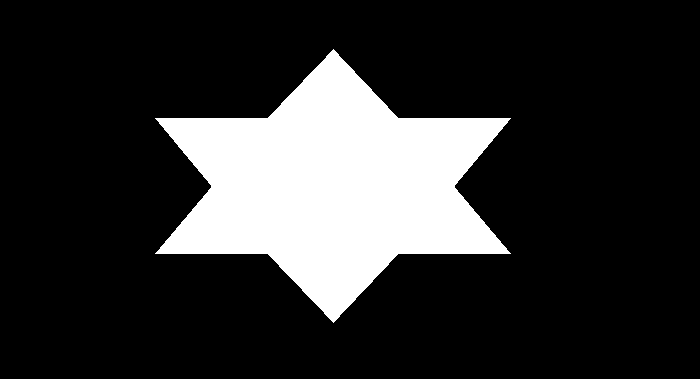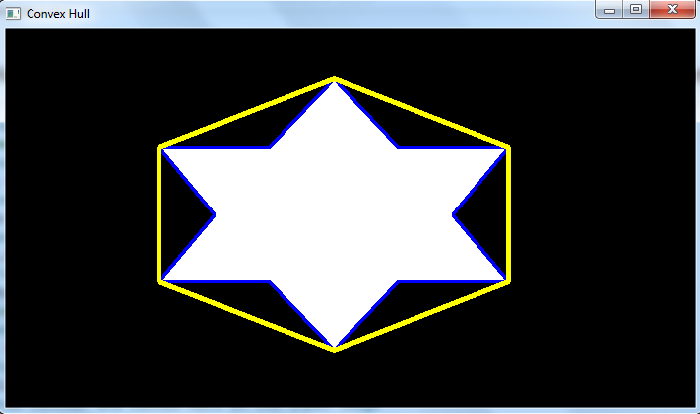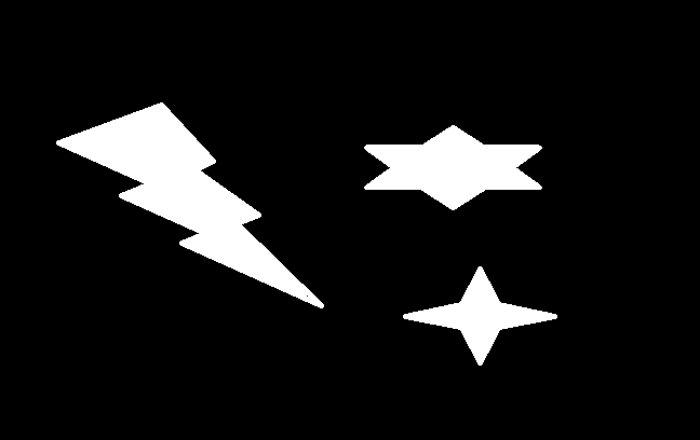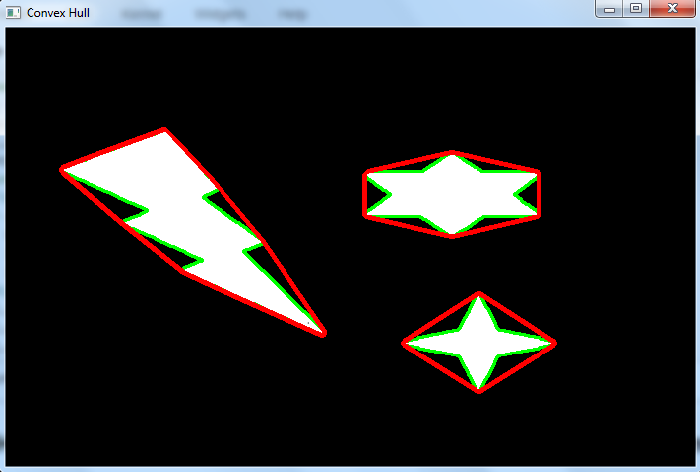# How to find and draw Convex Hull of an image contour in OpenCV Python?

A Convex Hull looks similar to contour approximation, but it is not exactly a contour approximation. A convex hull is a convex curve around an object. A convex curve is always bulged out, or at-least flat. A convex hull finds the convexity defects and corrects them.

## Syntax

To find the convex hull, we use the following function −

hull = cv2.convexHull(cnt, hull, clockwise, returnPoints)


## Arguments

• cnt are the contour points. It is represented as an array of contour points.

• hull is the output, normally we avoid it.

• clockwise − Orientation flag. If it is True, the output convex hull is oriented clockwise else it is oriented counter-clockwise.

• returnPoints − The default is set to True.

Output − When returnPoints is set to True it returns the coordinates of the hull points. If it is set to False, it returns the indices of contour points corresponding to the hull points.

So, to get a convex hull, we generally use the following −

hull = cv2.convexHull(cnt)


To draw the contours of the hull points, use this function −

cv2.drawContours(img, [hull], -1, (0,255,255), 3)


## Steps

You can use the following steps to find and draw the convex hull of an image contour −

Import the required library. In all the following Python examples, the required Python library is OpenCV. Make sure you have already installed it.

import cv2


img = cv2.imread('shape.png')
gray = cv2.cvtColor(img, cv2.COLOR_BGR2GRAY)


Apply thresholding on the grayscale image to create a binary image.

ret,thresh = cv2.threshold(gray,150,255,0)


Find the contours in the image using cv2.findContours() function.

contours, _ = cv2.findContours(thresh, cv2.RETR_TREE,cv2.CHAIN_APPROX_SIMPLE)


Select a contour cnt or loop over the all contours. Find the Convex Hull for the contour cnt using cv2.convexHull(cnt) function.

cnt = contours
hull = cv2.convexHull(cnt)


Draw the Convex Hull on the input image passing hull points to the below function.

cv2.drawContours(img, [hull], -1, (0,255,255), 3)


Display the image with the drawn Convex Hull.

cv2.imshow("Convex Hull", img)
cv2.waitKey(0)
cv2.destroyAllWindows()


Let's have a look at some examples for a better understanding.

## Example 1

In the Python program below, we detect the contours in the image and find the Convex Hull for the first contour. We also draw the first contour and Convex Hull on the image.

# import required libraries
import cv2

# convert the input image to grayscale
gray = cv2.cvtColor(img, cv2.COLOR_BGR2GRAY)

# apply thresholding to convert grayscale to binary image
ret,thresh = cv2.threshold(img1,150,255,0)

# find the contours
contours,hierarchy = cv2.findContours(thresh, cv2.RETR_TREE,cv2.CHAIN_APPROX_SIMPLE)
print("Number of contours detected:", len(contours))

# select the first contour
cnt = contours

# find the convex hull using the contour
hull = cv2.convexHull(cnt)

# draw contour and convex hull on the input image
img = cv2.drawContours(img,[cnt],0,(255,0,0),2)
img = cv2.drawContours(img,[hull],0,(0,255,255),3)

# display the image with drawn contour and convex hull
cv2.imshow("Convex Hull", img)
cv2.waitKey(0)
cv2.destroyAllWindows()


We will use the following image as the Input File in the above program code.## Output

When you execute the above code, it will produce the following output

Number of contours detected: 1


And we get the following output window, showing the first detected contour and Convex Hull in the image −The contour is drawn in blue color and the convex hull is drawn in yellow color. Notice the difference between the contour and convex hull.

## Example 2

In this example, we detect the contours in the image and find the convex hulls for the all contours. We also draw all the contours and convex hulls on the image.

import cv2
gray = cv2.cvtColor(img, cv2.COLOR_BGR2GRAY)

ret,thresh = cv2.threshold(gray,100,255,0)
contours,hierarchy = cv2.findContours(thresh, cv2.RETR_TREE,cv2.CHAIN_APPROX_SIMPLE)
print("Number of contours detected:", len(contours))

# Find the convex hull for all the contours
for cnt in contours:
hull = cv2.convexHull(cnt)
img = cv2.drawContours(img,[cnt],0,(0,255,0),2)
img = cv2.drawContours(img,[hull],0,(0,0,255),3)

# Display the image with convex hull drawn on it
cv2.imshow("Convex Hull", img)
cv2.waitKey(0)
cv2.destroyAllWindows()


We will use this image as the Input File in this program −## Output

When you execute the above code, it will produce the following output

Number of contours detected: 3


And we get the following output window, showing the detected contours and convex hulls in the image −The convex hulls are shown in red color and the contours are shown in the green color. Notice the difference between the contours and convex hulls.